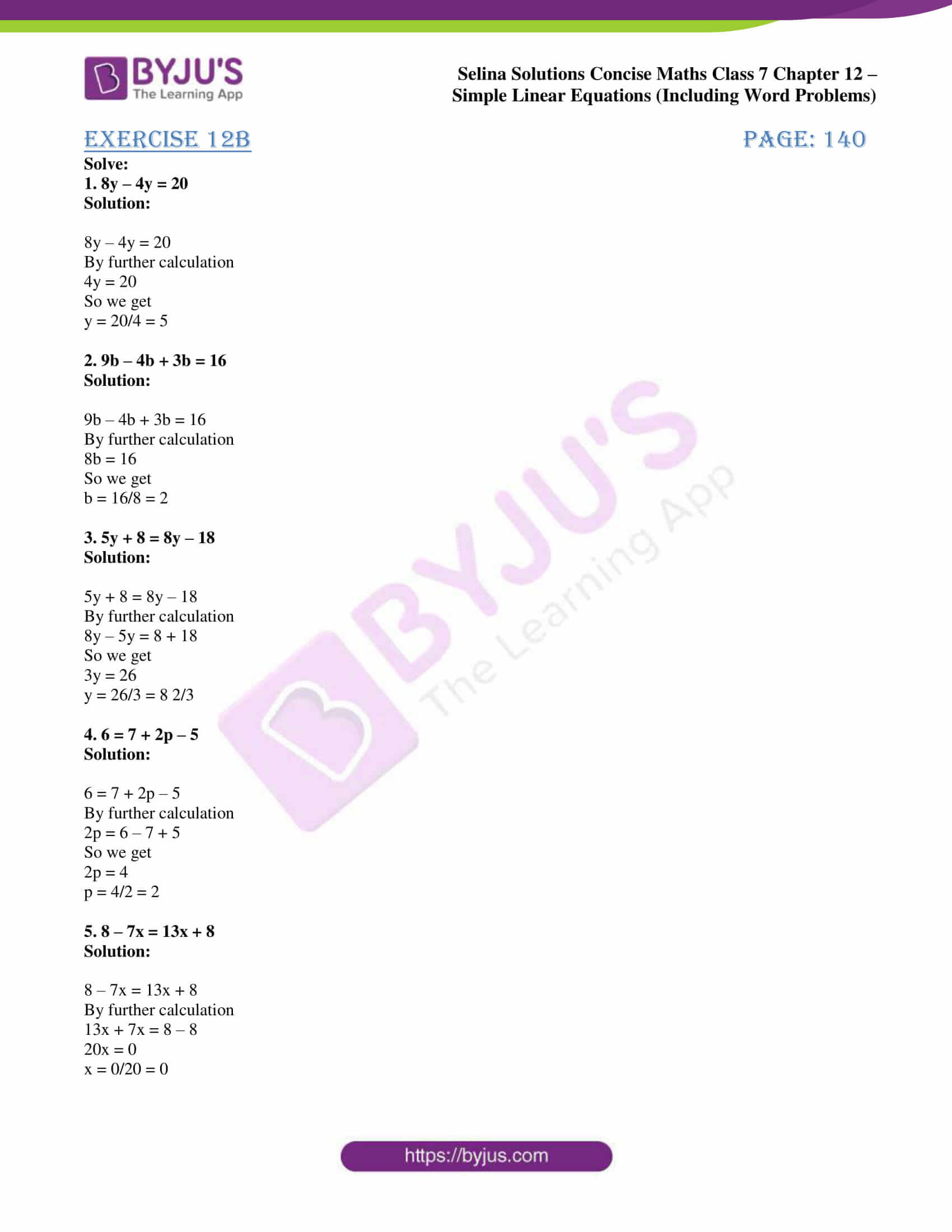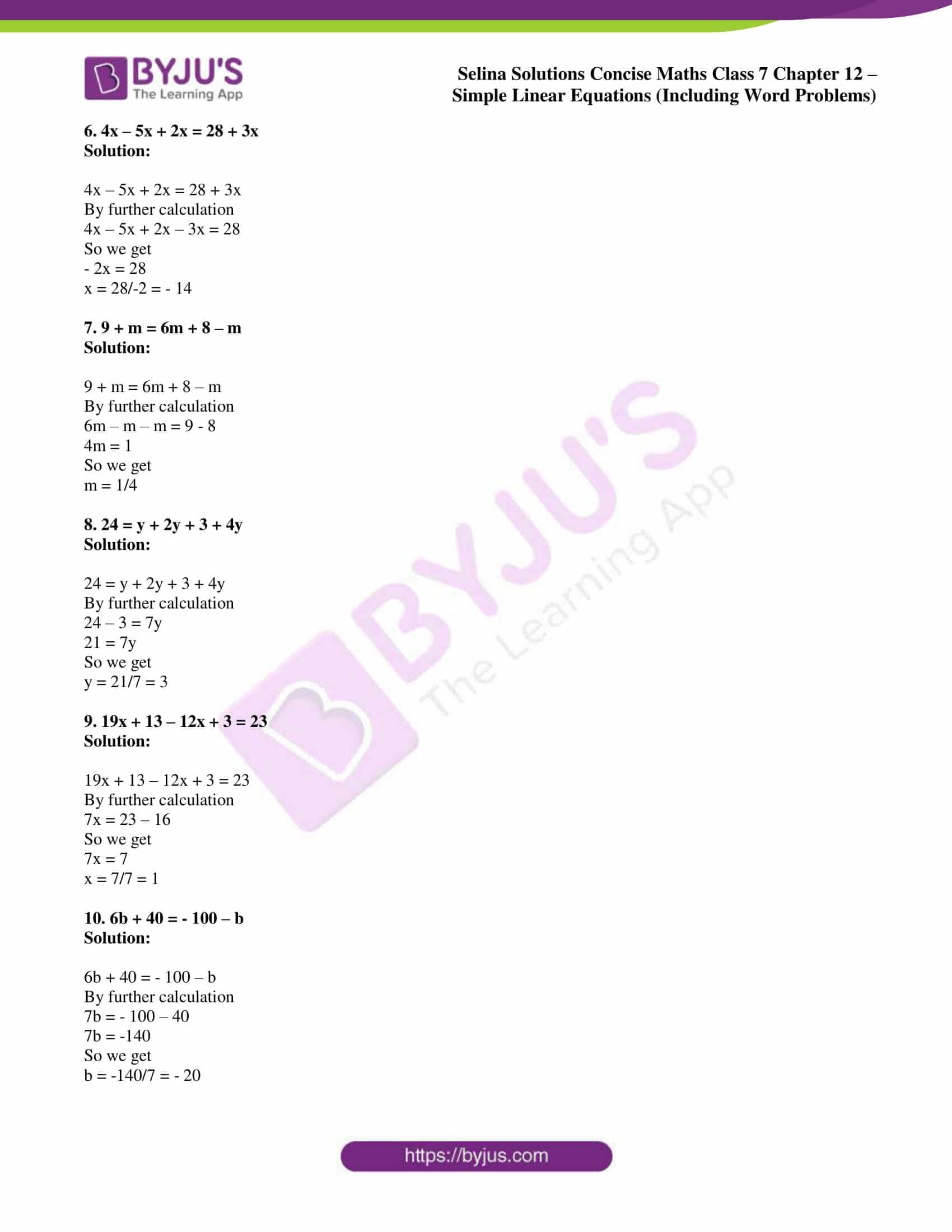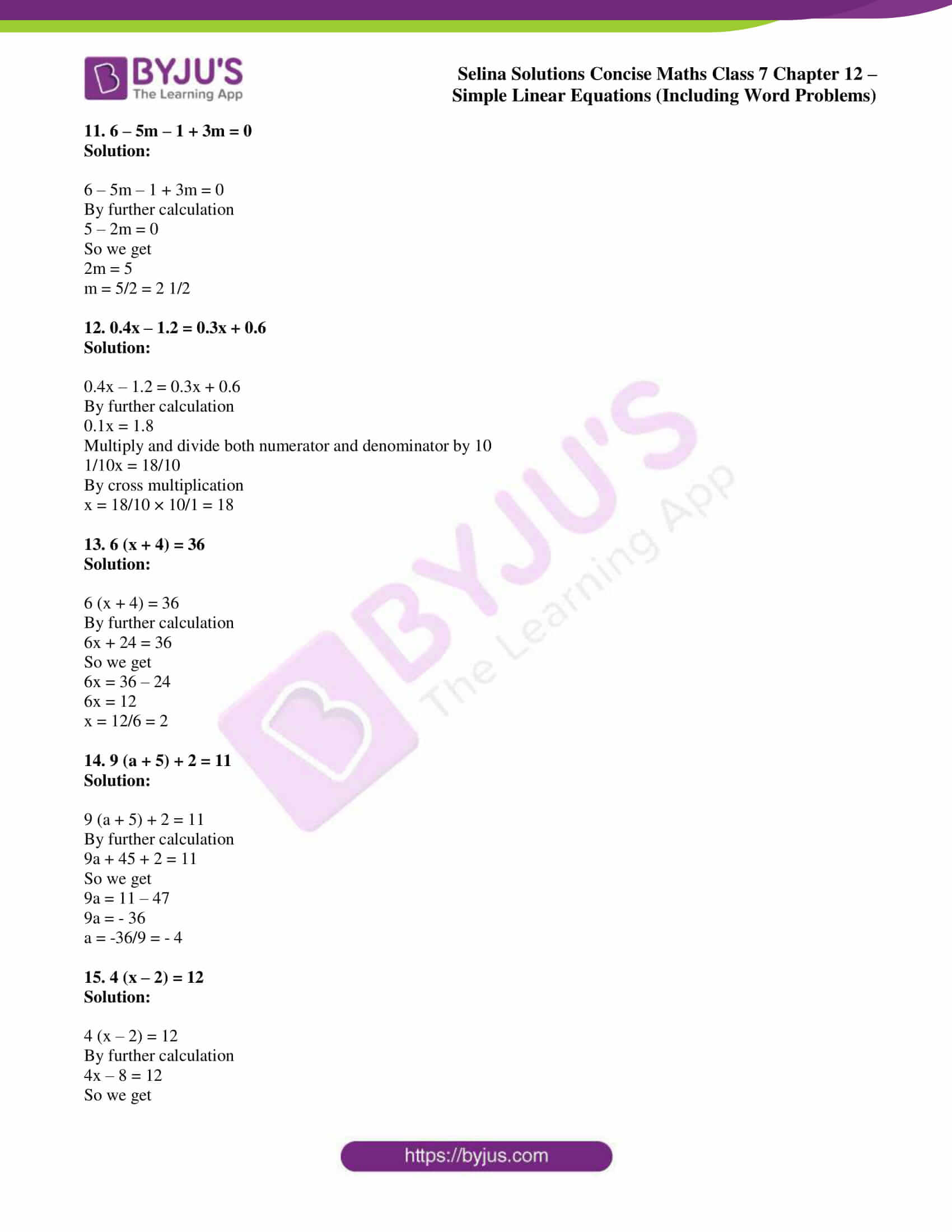# Selina Solutions Concise Maths Class 7 Chapter 12: Simple Linear Equations (Including Word Problems) Exercise 12B

Selina Solutions Concise Maths Class 7 Chapter 12 Simple Linear Equations (Including Word Problems) Exercise 12B is prepared with the aim of helping students perform well in the annual exam. The concept of solving an equation with the variable on both sides is covered under this exercise. The solutions PDF improves problem-solving and analytical skills among students. Students who aspire to score well in the annual exam can use Selina Solutions Concise Maths Class 7 Chapter 12 Simple Linear Equations (Including Word Problems) Exercise 12B, PDF from the links which are provided here.

## Selina Solutions Concise Maths Class 7 Chapter 12: Simple Linear Equations (Including Word Problems) Exercise 12B Download PDF### Access other exercises of Selina Solutions Concise Maths Class 7 Chapter 12: Simple Linear Equations (Including Word Problems)

Exercise 12A Solutions

Exercise 12C Solutions

Exercise 12D Solutions

### Access Selina Solutions Concise Maths Class 7 Chapter 12: Simple Linear Equations (Including Word Problems) Exercise 12B

#### Exercise 12B page: 140

Solve:

1. 8y – 4y = 20

Solution:

8y – 4y = 20

By further calculation

4y = 20

So we get

y = 20/4 = 5

2. 9b – 4b + 3b = 16

Solution:

9b – 4b + 3b = 16

By further calculation

8b = 16

So we get

b = 16/8 = 2

3. 5y + 8 = 8y – 18

Solution:

5y + 8 = 8y – 18

By further calculation

8y – 5y = 8 + 18

So we get

3y = 26

y = 26/3 = 8 2/3

4. 6 = 7 + 2p – 5

Solution:

6 = 7 + 2p – 5

By further calculation

2p = 6 – 7 + 5

So we get

2p = 4

p = 4/2 = 2

5. 8 – 7x = 13x + 8

Solution:

8 – 7x = 13x + 8

By further calculation

13x + 7x = 8 – 8

20x = 0

x = 0/20 = 0

6. 4x – 5x + 2x = 28 + 3x

Solution:

4x – 5x + 2x = 28 + 3x

By further calculation

4x – 5x + 2x – 3x = 28

So we get

– 2x = 28

x = 28/-2 = – 14

7. 9 + m = 6m + 8 – m

Solution:

9 + m = 6m + 8 – m

By further calculation

6m – m – m = 9 – 8

4m = 1

So we get

m = 1/4

8. 24 = y + 2y + 3 + 4y

Solution:

24 = y + 2y + 3 + 4y

By further calculation

24 – 3 = 7y

21 = 7y

So we get

y = 21/7 = 3

9. 19x + 13 – 12x + 3 = 23

Solution:

19x + 13 – 12x + 3 = 23

By further calculation

7x = 23 – 16

So we get

7x = 7

x = 7/7 = 1

10. 6b + 40 = – 100 – b

Solution:

6b + 40 = – 100 – b

By further calculation

7b = – 100 – 40

7b = -140

So we get

b = -140/7 = – 20

11. 6 – 5m – 1 + 3m = 0

Solution:

6 – 5m – 1 + 3m = 0

By further calculation

5 – 2m = 0

So we get

2m = 5

m = 5/2 = 2 1/2

12. 0.4x – 1.2 = 0.3x + 0.6

Solution:

0.4x – 1.2 = 0.3x + 0.6

By further calculation

0.1x = 1.8

Multiply and divide both numerator and denominator by 10

1/10x = 18/10

By cross multiplication

x = 18/10 × 10/1 = 18

13. 6 (x + 4) = 36

Solution:

6 (x + 4) = 36

By further calculation

6x + 24 = 36

So we get

6x = 36 – 24

6x = 12

x = 12/6 = 2

14. 9 (a + 5) + 2 = 11

Solution:

9 (a + 5) + 2 = 11

By further calculation

9a + 45 + 2 = 11

So we get

9a = 11 – 47

9a = – 36

a = -36/9 = – 4

15. 4 (x – 2) = 12

Solution:

4 (x – 2) = 12

By further calculation

4x – 8 = 12

So we get

4x = 20

x = 20/4 = 5